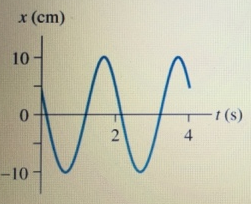# Problem: For the graph in (Figure 1),A. determine the frequency f. B. Determine the oscillation amplitude

###### FREE Expert Solution

Frequency:

$\overline{){\mathbf{f}}{\mathbf{=}}\frac{\mathbf{1}}{\mathbf{T}}}$

A.

The period, T is the time taken for one oscillation.

93% (164 ratings)###### Problem DetailsFor the graph in (Figure 1),

A. determine the frequency f.

B. Determine the oscillation amplitude